### 14.2.2.1 Reachability graph

After time discretization has been performed, the reachable set can be adapted to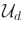to obtain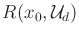. An interesting question is: What is the effect of sampling on the reachable set? In other words, how do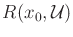anddiffer? This can be addressed by defining a reachability graph, which will be revealed incrementally by a planning algorithm.

Let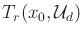denote a reachability tree, which encodes the set of all trajectories from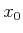that can be obtained by applying trajectories in. Each vertex ofis a reachable state,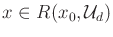. Each edge ofis directed; its source represents a starting state, and its destination represents the state obtained by applying a constant actionover time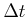. Each edge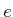represents an action trajectory segment,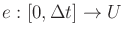. This can be transformed into a state trajectory,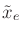, via integration using (14.1), from 0 toof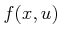from the source state of.

Thus, in terms of,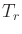can be considered as a topological graph in(will be used as an abbreviation of). The swath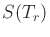ofis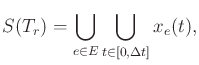(14.8)

in which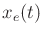denotes the state obtained at time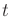from edge. (Recall topological graphs from Example 4.6 and the swath from Section 5.5.1.)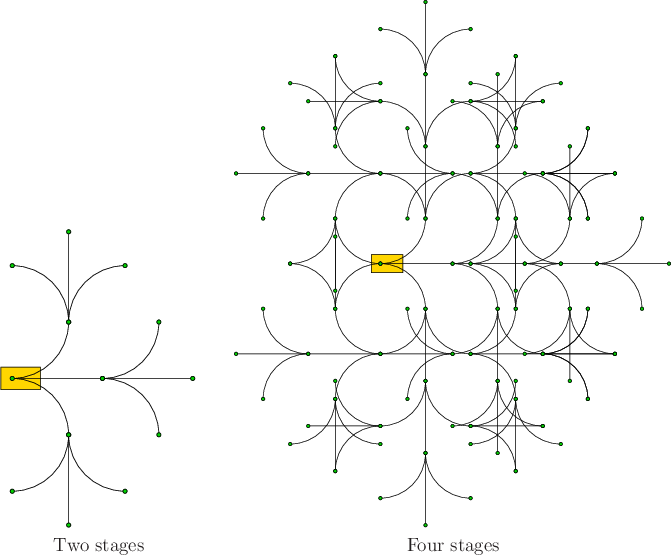Example 14..4 (Reachability Tree for the Dubins Car)   Several stages of the reachability tree for the Dubins car are shown in Figure 14.6. Suppose that there are three actions (straight, right-turn, left-turn), andis chosen so that if the right-turn or left-turn action is applied, the car travels enough to rotate by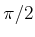. After the second stage, there are nine leaves in the tree, as shown in Figure 14.6a. Each stage produces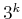new leaves. In Figure 14.6b,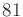new leaves are added in stage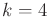, which yields a total of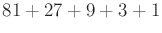vertices. In many cases, the same state is reachable by different action sequences. The swath after the first four stages is the set of all points visited so far. This is a subset ofthat is the union of all vertices and all points traced out byfor each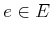.From Example 14.4 it can be seen that it is sometimes possible to arrive at the same state using two or more alternative action trajectories. Since each action trajectory can be expressed as an action sequence, the familiar issue arises from classical AI search of detecting whether the same state has been reached from different action sequences. For some systems, the reachability problem can be dramatically simplified by exploiting this information. If the same state is reached from multiple action sequences, then only one vertex needs to be represented.

This yields a directed reachability graph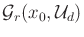, which is obtained fromby merging its duplicate states. If every action sequence arrives at a unique state, then the reachability graph reduces to the reachability tree. However, if multiple action sequences arrive at the same state, this is represented as a single vertex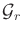. From this point onward, the reachability graph will be primarily used. As for a reachability tree, a reachability graph can be interpreted as a topological graph in, and its swath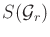is defined by adapting (14.8).

The simplest case of arriving at the same state was observed in Example 2.1. The discrete grid in the plane can be modeled using the terminology of Chapter 13 as a system of the form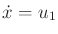and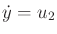for a state space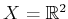. The discretized set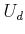of actions is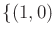,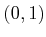,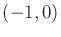,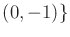. Let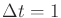. In this case, the reachability graph becomes the familiar 2D grid. If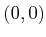is the initial state, then the grid vertices consist of all states in which both coordinates are integers.

Through careless discretization of an arbitrary system, such a nice grid usually does not arise. However, in many cases a discretization can be carefully chosen so that the states become trapped on a grid or lattice. This has some advantages in sampling-based planning. Section 14.4.1 covers a method that exploits such structure for the system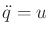. It can even be extended to more general systems, provided that the system can be expressed as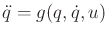and it is not underactuated. It was shown recently that by a clever choice of discretization, a very large class of nonholonomic systems14.4 can also be forced onto a lattice . This is usually difficult to achieve, and under most discretizations the vertices of the reachability graph are dense in the reachable set.

It is also possible to define backward versions of the reachability tree and reachability graph, in the same way that backward reachable sets were obtained. These indicate initial states and action sequences that will reach a given goal state and are no more difficult to define or compute than their forward counterparts. They might appear more difficult, but keep in mind that the initial states are not fixed; thus, no BVP appears. The initial states can be obtained by reverse-time integration of the state transition equation; see Section 14.3.2.

Steven M LaValle 2020-08-14